# ILP Part 36 — Various goal types

This is the thirty sixth part of the ILP series. For your convenience you can find other parts in the table of contents in Part 1 – Boolean algebra

Today we will try to introduce various goal types to our problems. There are six main types of optimizations: maximizing, minimizing, maximizing maximum, maximizing minimum, minimizing maximum, and minimizing minimum. Let’s go.

# Maximizing and minimizing

These two are pretty easy. First of all, our solver needs to be able to optimize our cost function, usually minimize it. Imagine that we have minimizing of function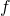out of the box. In order to maximize this function, we simply need to negate it: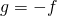. It also works the other way round: if we have maximizing in our solver, we can negate function to have minimization problem.

# Maximize minimum and minimize maximum

These two looks difficult but are in fact very easy. Let’s assume, that we are able to maximize cost function. We have variables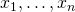and we want to maximize minimum of them. We could do something like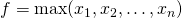since we already know how to calculate maximum of variables, but there is a better way. We introduce another variableand add the following constraints: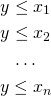And now we maximize function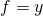. How does it work? Well, sincemust be less or equal than all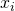, solver needs to take care of fulfilling these constraints. At the same time, solver has to maximize goal function which will indirectly maximize minimum of variables.

Minimizing maximum works the same way: we introduce another variable, add constraints (this time “greater or equal”) and minimize the variable.

# Maximize maximum and minimize minimum

These two goals are difficult because there is no shortcut. Let’s take first one: maximizing maximum. We have variable. We introduce another variableand try to match it with one of the other variables: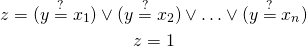And now we maximize. Minimizing minimum works the same way.

# Summary

If we constrain variable just to use it in cost function, it is usually possible to use some clever tricks to calculate result. For instance, when maximizing minimum we could just calculate minimum of variables (usingoperation) and maximize the result, but it would be much more computationally expensive. Always look for trick which will reduce size of your problem.